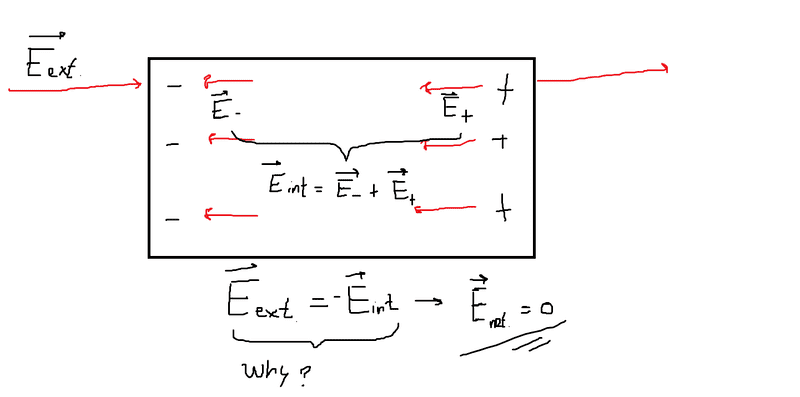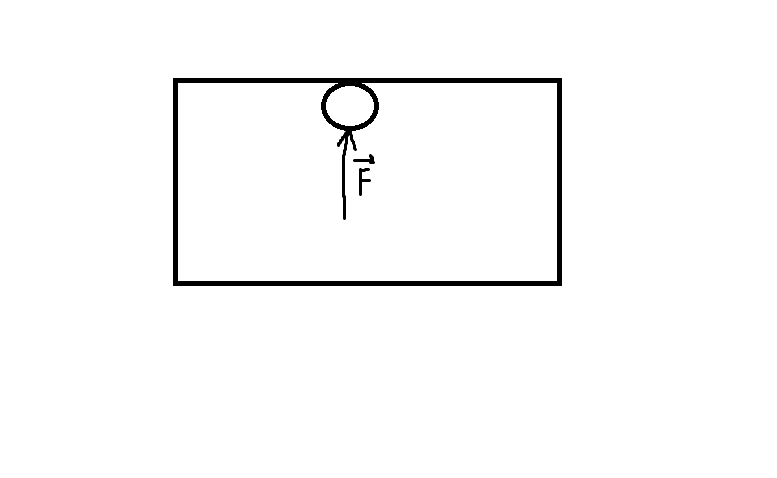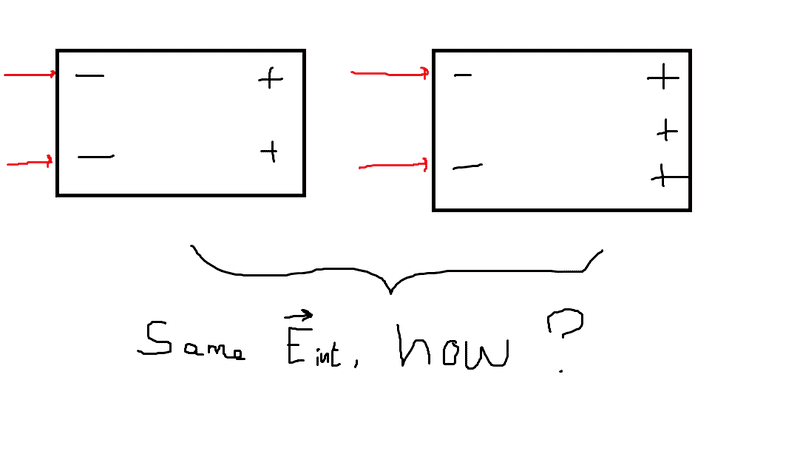# Electric field inside a conductor

APUGYael
The electric field inside a conductor is zero. Or: the internal electric field is equal in magnitude but opposite in direction to the external electric field, so that the net electric field inside the conductor is zero.

Why is this? The part where I am confused is why the magnitude is equal.

Is it just that we measured the electric field inside to be zero, and so concluded that the internal electric field should oppose the external electric field?Bonus question: What would happen to a particle (of an object) near the surface if you put a force on it pointing outward of the object. Would it jump out?Looking forward to a response.

-Y

#### Attachments

Gold Member
The part where I am confused is why the magnitude is equal.
Once this conductor has reached equilibrium, (a very important condition) there is no more flow of charges so the Forces on the charges (i.e. Fields) must be zero.
This doesn't apply when there is a current flowing in a real wire. In a circuit with real wires, there are very small fields causing the charge (sometimes very large) to flow but we mostly can ignore this because we would usually choose THICK enough wire.

APUGYael
Once this conductor has reached equilibrium, (a very important condition) there is no more flow of charges so the Forces on the charges (i.e. Fields) must be zero.
This doesn't apply when there is a current flowing in a real wire. In a circuit with real wires, there are very small fields causing the charge (sometimes very large) to flow but we mostly can ignore this because we would usually choose THICK enough wire.
Right.
Once this conductor has reached equilibrium, (a very important condition) there is no more flow of charges so the Forces on the charges (i.e. Fields) must be zero.
This doesn't apply when there is a current flowing in a real wire. In a circuit with real wires, there are very small fields causing the charge (sometimes very large) to flow but we mostly can ignore this because we would usually choose THICK enough wire.

Right.

So why is it then that any extra positive charge added to the conductor creates an internal electric field that is STILL equal in magnitude to the external electric field. Wouldn't that charge create an internal electric field that is larger in magnitude than the external electric field?

I'm obviously missing something here. Is my concept of electric fields just off, or..?

-Y

Gold Member
The internal field will be the gradient of the Potential in the conductor, which is zero. Adding an unbalanced charge will just add a Potential to the whole conductor but it will not alter the gradient (i.e. it will not produce an internal Field).
Alternatively, there still can be no internal field or charges will flow. Current will flow briefly but, once equilibrium is established there will be no imbalanced charges and no Fields.

Gold Member
So why is it then that any extra positive charge added to the conductor creates an internal electric field that is STILL equal in magnitude to the external electric field.
This doesn't make sense. There will be no internal field or charges would be flowing. How can they and when would they stop?

APUGYael
This doesn't make sense. There will be no internal field or charges would be flowing. How can they and when would they stop?

As I pointed out earlier: for a conductor with net charge ZERO the internal electric field is opposite in direction to the external electric field but equal in magnitude. The net electric field inside the conductor is therefore ZERO (simple vector sum).

If we repeat the same thing but this time with a conductor that has a net charge that is non-zero (let's say: +Q), the process repeats:
1. Conductor becomes induced (-Q on one side, +2Q on the other side)
2. Electric field inside conductor opposes external electric field (same magnitude, opposite direction)
3. And so: there is no net electric field inside the box

Note that I did not make any changes to the external electric field. It's still the same one as I used on the conductor with ZERO net charge.

That is completely nonsensical to me. An increase in net charge in a conductor doesn't add to the internal electric field of the conductor.

I understand the reasoning: no movement -> no net force -> equilibrium -> net electric field must be zero.

But how can charges rearrange themselves in such a way that they cancel out the external electric field? Book says: "If there were an electric field, the electrons would move until they reached positions where the electric field, and therefore the electric force on them, did become zero". What does this arrangement look like?I hope I'm making some sense here.

#### Attachments

Last edited:
Mentor
What does this arrangement look like?
Your picture is misleading you. If the added charge were a single charged particle that had to sit at one point, then you'd be right - no matter where you put it, you won't be able to keep the internal field zero everywhere. But when you're using this model of an ideal conductor, you're assuming that the charge is carried by a very large number of particles each carrying a very small amount of charge, so that the charge willl be distributed smoothly (think about how the surface of a cup of water will be smooth and level even though the water is made up of particles, but you can't do the same thing with a cup of gravel).

So back to your conductor again. The charge carriers have distributed themselves in such a way that they cancel the field from outside, and then you add some more charge. Consider how that additional charge would behave if you didn't have that external field - it would smoothly distribute itself along the surface of the perimeter of the conductor in such a way that it produces no internal field. Now turn the external field back on again - what happens?

APUGYael
Your picture is misleading you. If the added charge were a single charged particle that had to sit at one point, then you'd be right - no matter where you put it, you won't be able to keep the internal field zero everywhere. But when you're using this model of an ideal conductor, you're assuming that the charge is carried by a very large number of particles each carrying a very small amount of charge, so that the charge willl be distributed smoothly (think about how the surface of a cup of water will be smooth and level even though the water is made up of particles, but you can't do the same thing with a cup of gravel).

So back to your conductor again. The charge carriers have distributed themselves in such a way that they cancel the field from outside, and then you add some more charge. Consider how that additional charge would behave if you didn't have that external field - it would smoothly distribute itself along the surface of the perimeter of the conductor in such a way that it produces no internal field. Now turn the external field back on again - what happens?

Simply adding a net charge to the conductor produces an electric field, which forces the particles to rearrange themselves in such a way that there is no electric field. I like to think of this as nature seeking balance.

But then:
Why do uniformly distributed charges not create a net electric field, no matter how many charges there are (i.e. why does the internal electric field of a conductor not rely on the amount of charges)?

I get what you ment with the water and gravel. But surely adding charges, even if distributed uniformly & splitting the total charge added up into a lot of tiny charges, would affect the magnitude of the overall electric field. Are the resultant field lines of the internal electric field for both situations (conductor net charge 0 and conductor net charge +Q) the same?

Last edited:
MDya
Hi!
4 years later -- and you're not alone: I have the same doubts about the overall magnitude.
My question is: can there be a situation when the internal field of a conductor is bigger in magnitude than the external one?
Say, we moved further away the two plates of a capacitor, so now the force between them is smaller. What'll happen to the particles of the conductor? How can they still be in equilibrium if the outter force had lost in the magnitude whereas the force of the internal field is still the same?

Gold Member
2022 Award
If I understand it right you discuss electrostatics, i.e., everything is time independent and there are no stationary currents. Then ##\vec{B}=0## everywhere and in the interior of the conductor is also ##\vec{E}=0##. If the conductor carries a non-zero net charge, then this charge is distributed over the surface of the conductor in such a way that there's no field inside the conductor, i.e., ##\rho=0## inside the conductor and all the net charge is distributed as a surface charge ##\sigma##.

To understand how this works, I recommend to carefully calculate the electrostatic field of an isolated charged sphere. For that purpose just solve ##\Delta \Phi=0## which is valid for the electrostatic potential, ##\Phi##, everywhere except on the surface. Due to symmetry ##\Phi=\Phi(r)##. It's just the boundary conditions you need to work out, but it's really easy.

Gold Member
My question is: can there be a situation when the internal field of a conductor is bigger in magnitude than the external one?
Does this question actually make sense, when you take into account the definitions of a conductor and an insulator (the air around it)? It could be interesting to consider a shape with one conductuctivity, fitted exactly inside a hollow shape with a different conductivity but your assumption of zero infinite conductivity for your two 'media' sort of begs the question.

Gold Member
2022 Award
For electrostatics conductivity is irrelevant. For magnetostatics it's of course an interesting problem to treat this problem.

•